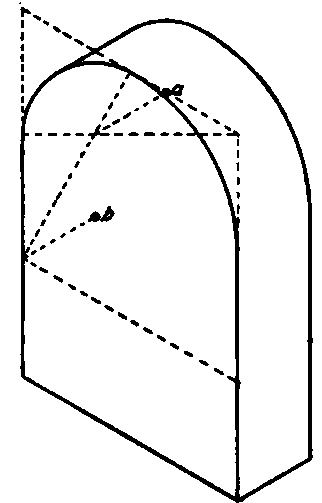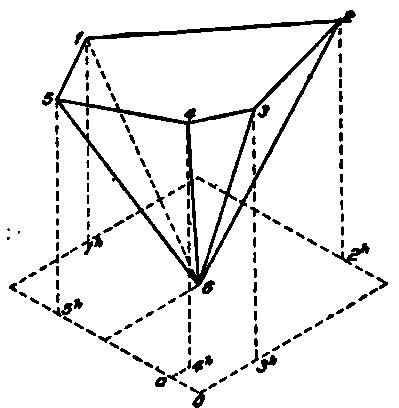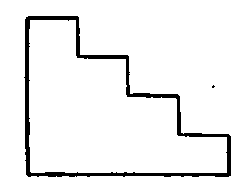Fig. 156 shows a cube with circles inscribed in the top and two side faces. The isometric of a circle is an ellipse, the exact construction of which would necessitate finding a number of points; for this reason an approximate construction by arcs of circles is often made. In the method, Fig. 156, four centers are used. Considering the upper face of the cube, lines are drawn from the obtuse angles f and e to the centers of the opposite sides. The intersections of these lines give points g and h, which serve as centers for the ends of the ellipse. With g as center and radius ga, the arc ad is drawn, and with f as center and radius fd, the arc dc is drawn; the ellipse is finished by using centers h and e. This construction is applied to all three faces.Fig. 156. Isometric of a Cube with Circles Inscribed on its Faces.Fig. 157. Isometric of a Cylinder.Fig. 158. Isometric of a Wooden Block.Fig. 159. Isometric of a Wooden Brace.

## Cylinder

Fig. 157 is the isometric of a cylinder standing on its base.

## Blocks

Fig. 158 represents a block with smaller blocks projecting from three faces.

## Framework

Fig. 159 shows a framework of three pieces, two at right angles and a slanting brace. The horizontal piece is mortised into the upright as indicated by the dotted lines.

## House

In Fig. 160 the isometric outline of a house is represented, showing a dormer window and a partial hip roof; a b is a hip rafter, cd a valley. Let the pitch of the main roof be shown at B, and let m be the middle point of the top of the end wall of the house. Then, by measuring vertically up a distance ml equal to the vertical height an shown at B, a point on the line of the ridge will be found at l. Line li is equal to b A, and i h is then drawn. Let the pitch of the end roof be given at A. This shows that the peak of the roof, or the end a of the ridge, will be back from the end wall a distance equal to the base of the triangle at A. Hence, lay off from I this distance, giving point a, and join a with b and x.

The height he of the ridge of the dormer roof is known, and it must be found where this ridge will meet the main roof. The ridge must be a 30-degree line as it runs parallel to the end wall of the house and to the ground. Draw from e a line parallel to b h to meet a vertical through h and f. This point is in the vertical plane of the end wall of the house, hence in the plane of ih. If now a 30-degree line be drawn from f parallel to x b, it will meet the roof of the house at g. The dormer ridge and fg are in the same horizontal plane, hence will meet the roof at the same distance below the ridge ai. Therefore draw the 30-degree line g c, and connect c with d.Fig. 160. Isometric Outline of a House.

## Box With Cover

In Fig. 161 a box is shown with the cover opened through 150 degrees. The right-hand edge of the bottom shows the width, the left-hand edge shows the length and the vertical edge shows the height. The short edges of the cover are not isometric lines, hence are not shown in their true lengths; neither is the angle through which the cover is opened represented in its actual size.

The corners of the cover must then be determined by co-ordinates from an end view of the box and cover. As the end of the cover is in the same plane as the end of the box, the simple end view as shown in Fig. 162 will be sufficient. Extend the top of the box to the right, and from e and d let fall perpendiculars on ab produced, giving the points e and f. The point c may be located by means of the two distances or coordinates be and ec, and these distances will appear in their true lengths in the isometric view. Hence produce a'b' to e' and f'; and from these points draw verticals e'c' and f'd'; make b'e' equal to be, e'c' equal to ec; and similarly for d'. Draw the lower edge parallel to c'd' and equal to it in length, and connect with 6'.Fig. 161. Isometric of a Box and Cover.Fig. 162. End View of Box Shown in Fig. 161.

It will be seen that in isometric drawing parallel lines always appear parallel. It is also true that lines divided proportionally maintain this same relation in isometric drawing.

## Prism With Semicircular Top

Fig. 163 shows a block or prism with a semicircular top. Find the isometric of the square circumscribing the circle, then draw the curve by the approximate method. The centers for the back face are found by projecting the front centers back 30 degrees equal to the thickness of the prism, as shown at a and b.Fig. 163. Isometric of a Priam with Semicircular Top.Fig. 165. Isometric of Fig. 164.Fig. 164. Flan and Elevation of Oblique Pentagonal Pyramid.

### Pyramid

The plan and elevation of an oblique pentagonal pyramid are shown in Fig. 164. It is evident that none of the edges of the pyramid can be drawn in isometric as either vertical or 30-degree lines; hence a system of co-ordinates must be used as shown in Fig. 165. This problem illustrates the most general case; and to locate some of the points three co-ordinates must be used, two at 30 degrees and one vertical.

Circumscribe, about the plan of the pyramid, a rectangle which shall have its sides respectively parallel and perpendicular to a T-square line.

The isometric of this rectangle can be drawn at once with 30-degree lines, as shown in Fig. 165, o being the same point in both figures. The horizontal projection of point 3 is found in isometric at 3h, at the same distance from o as in the plan. That is, any distance which in plan is parallel to a side of the circumscribing rectangle, is shown in isometric in its true length and parallel to the corresponding side of the isometric rectangle. If point 3 were on the horizontal plane its isometric would be 3*, but the point is at the vertical height above H given in the elevation; hence, lay off above 3* this vertical height, obtaining the actual isometric of the point. To locate point 4, draw 4 a parallel to the side of the rectangle; they lay off oa and a 4h, giving what may be called the isometric plan of 4. The vertical height taken from the elevation locates the isometric of the point.Fig. 166. Isometric of a Skeleton of a Box.Fig. 167. Isometric of a Carpenter's Bench.Fig. 168. Plan and Elevation of Sawhorse.Fig. 169. Isometric of Fig. 168.

In like manner all the corners of the pyramid, including the apex, are located. The rule is, locate first in isometric the horizontal projection of a point by one or two 30-degree co-ordinates; then ver-tically above this point, locate its height as taken from the elevation. Figs. 166 to 173 give examples of the isometric of various objects.Fig. 170. End Elevation of Stairs.Fig. 171. Isometric of Stairs.

Fig. 168 is the plan and elevation, and Fig. 169 the isometric, of a carpenter's sawhorse.Fig. 172. Isometric of a Hollow Cylinder.Fig. 173. Isometric of a Wooden Model.